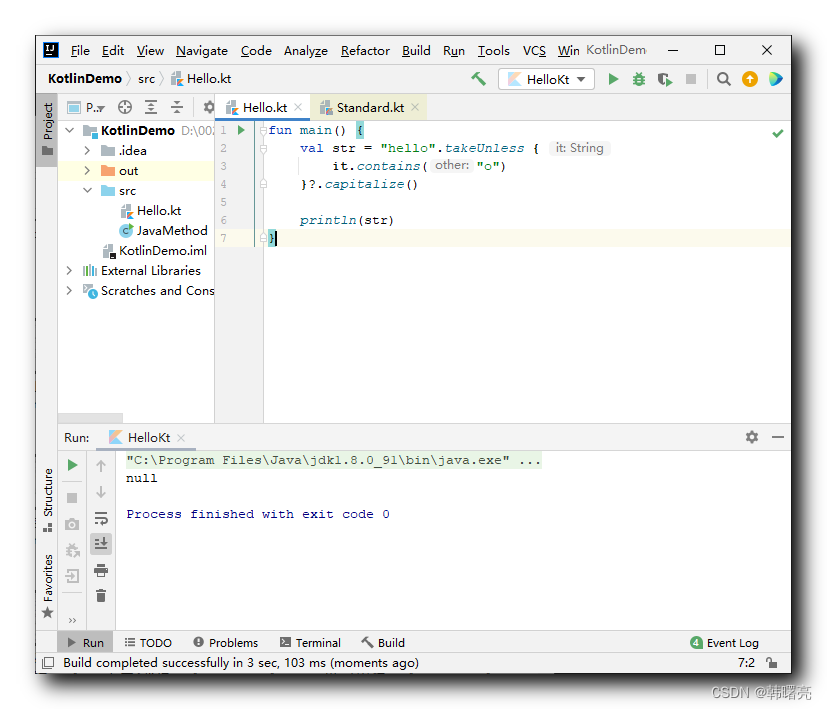## 【Kotlin】标准库函数总结 ( apply 函数 | let 函数 | run 函数 | with 函数 | also 函数 | takeIf 函数 | takeUnless 函数 )

### 文章目录

Kotlin 语言中 , 在 Standard.kt 源码中 , 为所有类型定义了一批标准库函数 , 所有的 Kotlin 类型都可以调用这些函数 ;

# 一、apply 标准库函数

Kotlin 标准库函数 中的 apply 函数 ,

apply 函数 的返回值 是 接收者对象 ,

apply 标准库函数原型 :

``````/**
* 以' this '值作为接收者调用指定函数[block]，并返回' this '值。
*
* 有关详细使用信息，请参阅[scope functions]的文档
* (https://kotlinlang.org/docs/reference/scope-functions.html#apply)。
*/
@kotlin.internal.InlineOnly
public inline fun <T> T.apply(block: T.() -> Unit): T {

contract {

callsInPlace(block, InvocationKind.EXACTLY_ONCE)
}
block()
return this
}
``````

``````import java.io.File

fun main() {

val file = File("hello.txt")
file.setWritable(true)
file.setExecutable(true)
}
``````

apply 函数代码示例 : 后面设置 可读 , 可写 , 可执行 权限的配置操作 , 可以在 apply 标准库函数中完成 , 代码如下 :

``````import java.io.File

fun main() {

val file = File("hello.txt").apply {

this.setWritable(true)
this.setExecutable(true)
}
}
``````

# 二、let 标准库函数

Kotlin 标准库函数 中的 let 函数 ,

apply 函数与 let 函数的区别 :

• apply 函数的 返回值是 调用者 ;
• let 函数的 返回值是 Lambda 表达式的最后一行 ;

let 函数原型 :

``````/**
* 调用以' this '值作为参数的指定函数[block]，并返回其结果。
*
* 有关详细使用信息，请参阅[scope functions]的文档
* (https://kotlinlang.org/docs/reference/scope-functions.html#let)。
*/
@kotlin.internal.InlineOnly
public inline fun <T, R> T.let(block: (T) -> R): R {

contract {

callsInPlace(block, InvocationKind.EXACTLY_ONCE)
}
return block(this)
}
``````

``````fun main() {

val name = "tom".let {

it.capitalize()
}
println(name)
}
``````

let 函数返回的是 匿名函数 的最后一行 , 因此将 “Tom” 字符串 返回了 ;

``````Tom
``````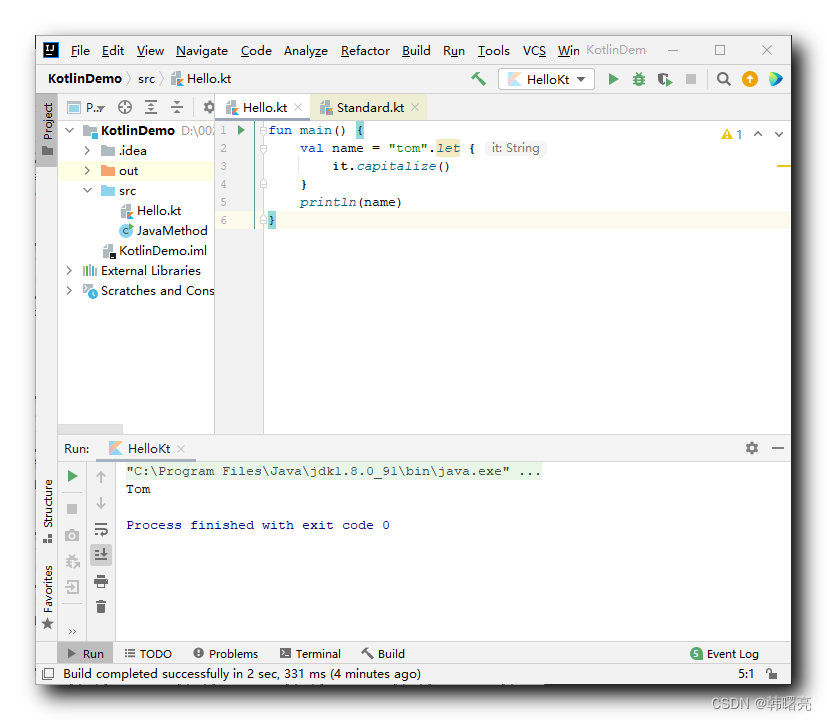let 函数与 空安全操作符 `?.` , 空合并操作符 `?:` 结合使用 , 可替代 if 语句效果 ;

``````fun main() {

val name: String? = null
println(getName(name))
}

fun getName(name: String?): String {

return name?.let {

"欢迎 \$name 同学"
}?: "name 为空"
}
``````

``````name 为空
``````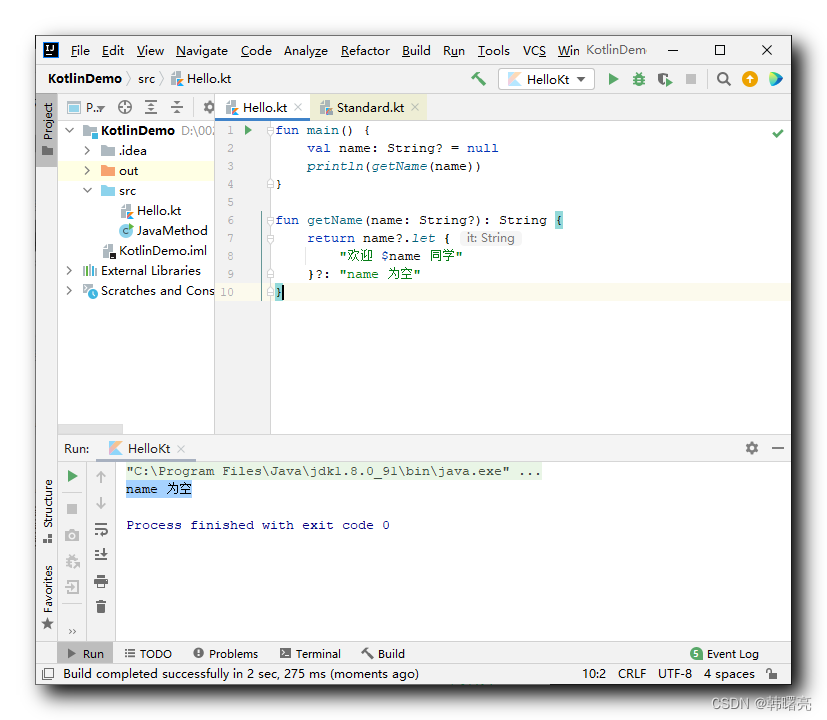# 三、run 标准库函数

## 1、run 函数传入 Lambda 表达式作为参数

run 标准库函数原型如下 :

``````/**
* 调用以' this '值为接收者的指定函数[block]，并返回结果。
*
* 有关详细使用信息，请参阅[scope functions]的文档(https://kotlinlang.org/docs/reference/scope-functions.html#run)。
*/
@kotlin.internal.InlineOnly
public inline fun <T, R> T.run(block: T.() -> R): R {

contract {

callsInPlace(block, InvocationKind.EXACTLY_ONCE)
}
return block()
}
``````

run 函数 传入 T.() -> R 类型 Lambda 表达式 作为参数 ,

run 函数的 Lambda 表达式参数 返回的是 boolean 类型的 true 值 ,

``````fun main() {

val ret = "Hello".run {

true
}
println(ret)
}
``````

``````true
``````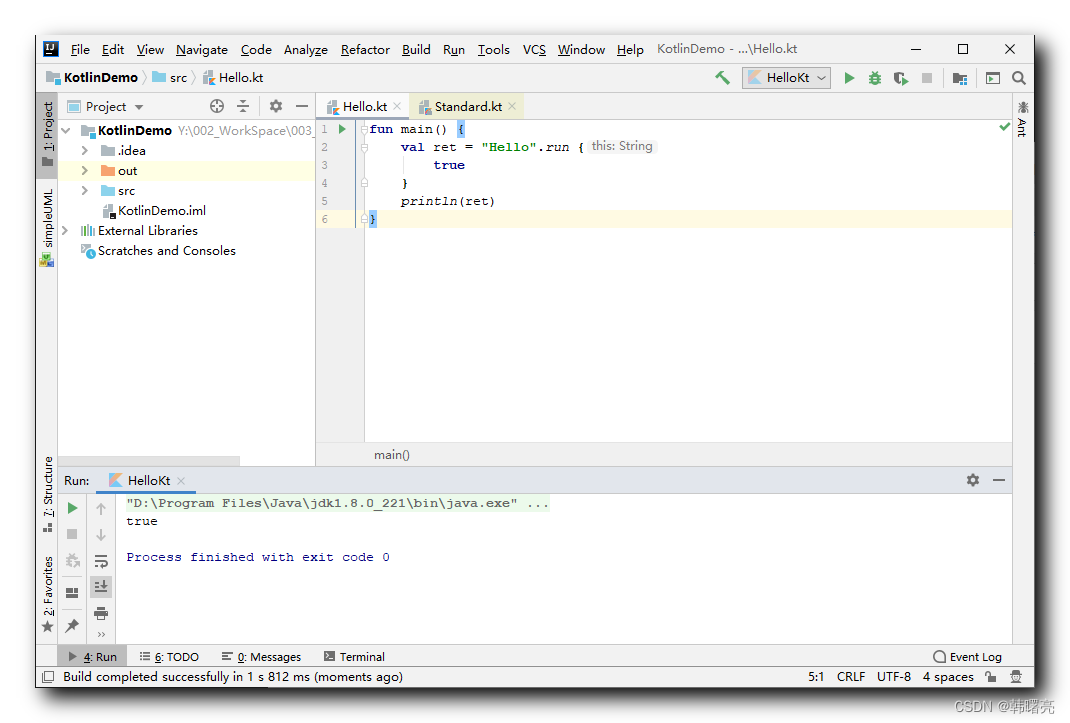## 2、run 函数传入函数引用作为参数

``````public inline fun <T, R> T.run(block: T.() -> R): R {

}
``````

run 函数 , 传入 T.() -> R 类型 函数参数 ,

`"hello".run(::hasO)` 代码 等价于 `hasO("hello")` 代码 ;

`"hello".run(::hasO).run(::log)` 代码 等价于 `log(hasO("hello"))` 代码 ;

`"hello".run(::hasO).run(::log).run(::println)` 代码 等价于 `println(log(hasO("hello")))` 代码 ;

``````fun main() {

"hello"
.run(::hasO)
.run(::log)
.run(::println)
}

fun hasO(name: String): Boolean {

return name.contains("o")
}

fun log(hasO: Boolean): String {

if (hasO) {

return "name has o"
} else {

return "name doesn't has o"
}
}
``````

``````name has o
``````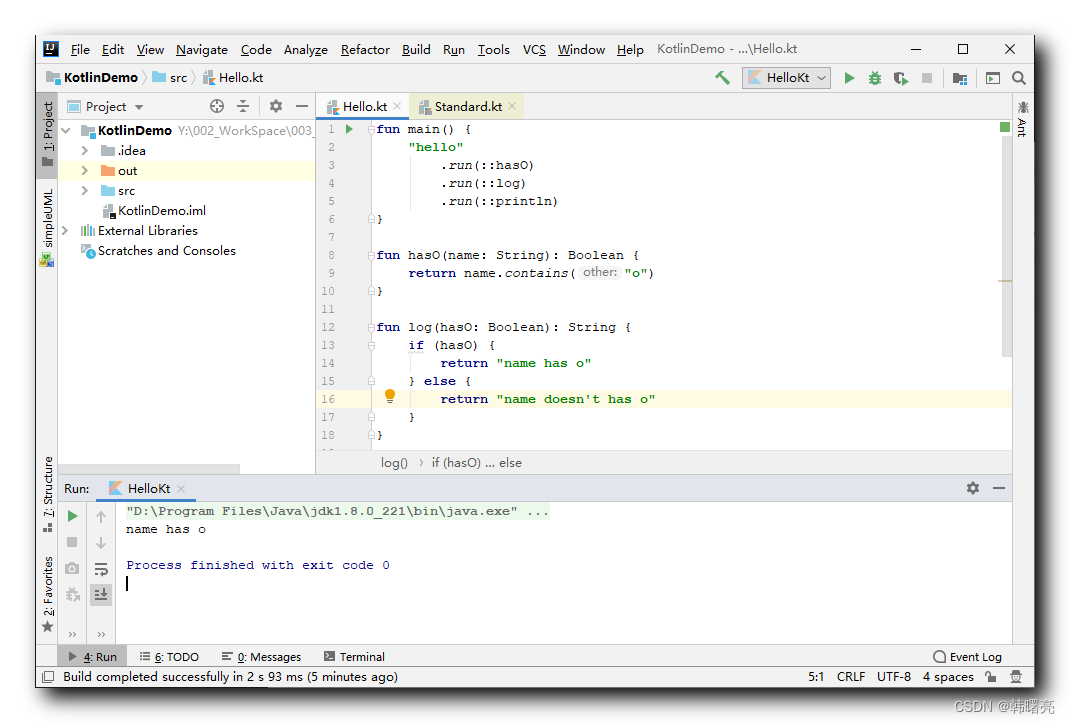# 四、with 标准库函数

with 函数run 函数 功能是一样的 ,

with 函数原型 :

``````/**
*
* 有关详细使用信息，请参阅[scope functions]的文档
* (https://kotlinlang.org/docs/reference/scope-functions.html#with)。
*/
@kotlin.internal.InlineOnly
public inline fun <T, R> with(receiver: T, block: T.() -> R): R {

contract {

callsInPlace(block, InvocationKind.EXACTLY_ONCE)
}
}
``````

with 函数的第一个参数是 receiver: T 接收者 ,

``````fun main() {

val str = with("hello") {

capitalize()
}
println(str)
}
``````

``````Hello
``````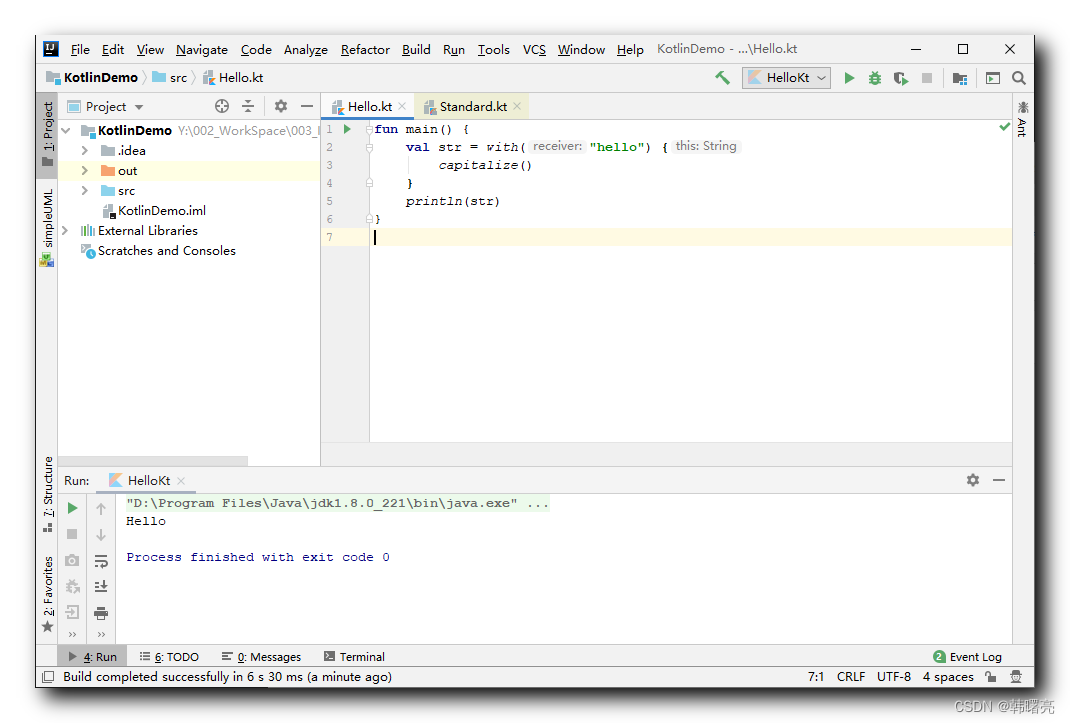``````fun main() {

val str = "hello".run {

capitalize()
}
println(str)
}
``````

``````Hello
``````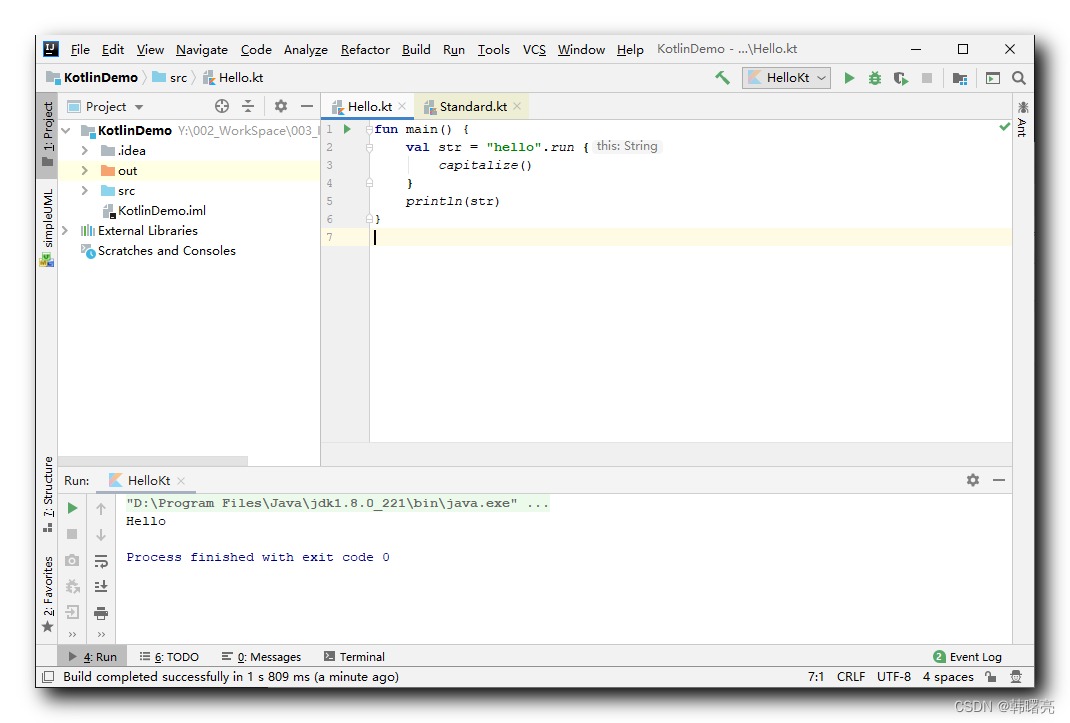# 五、also 标准库函数

also 函数 功能与 let 函数 功能 类似 ;

also 函数 将 接收者 ( 函数调用者 ) 作为参数传递给 Lambda 表达式参数 ,

also 函数 与 let 函数 返回值不同 ,

also 函数 返回 接收者对象本身 ,

let 函数 返回 Lambda 表达式的最后一行 ;

also 函数 返回 接收者对象本身 , 那么就可以使用该特性 , 对 接收者 执行 函数式编程的 链式调用 ;

``````fun main() {

val str = "hello".also {

println(it)
}.also {

// 该对象的生命周期仅限于该闭包
println(it.capitalize())
}

// 最终打印的是最初的 接收者对象
println(str)
}
``````

``````hello
Hello
hello
``````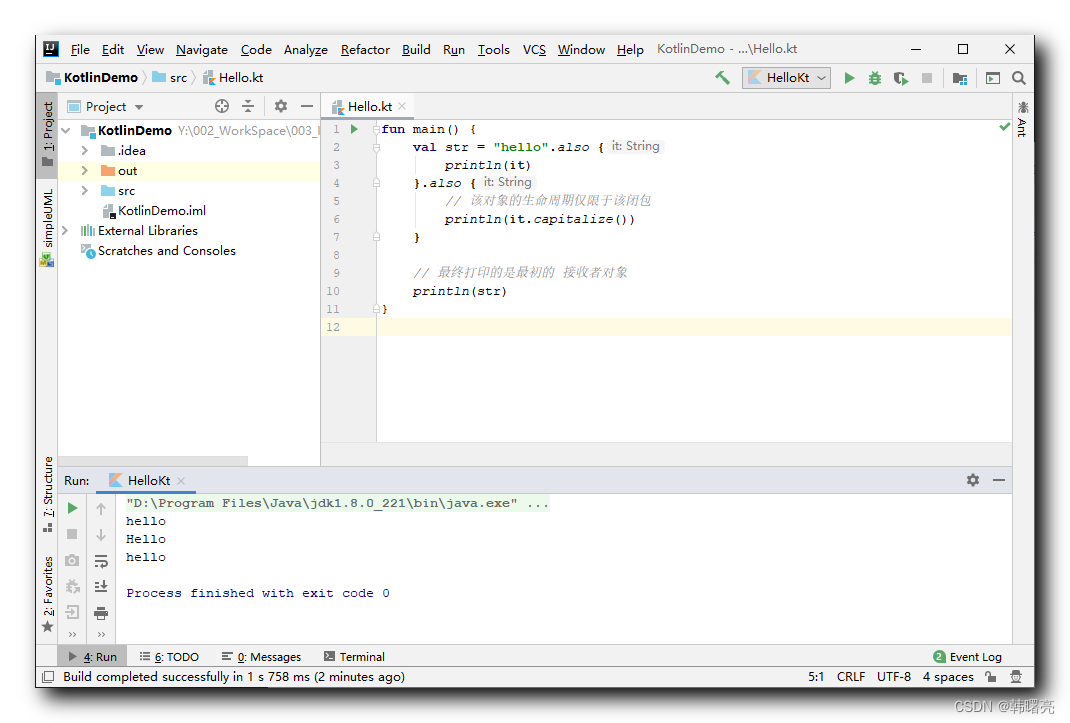# 六、takeIf 标准库函数

takeIf 函数 的 返回值 由其 Lambda 表达式参数的返回值 确定 ,

Lambda 表达式 返回 true , 则 返回 接收者对象 ;

Lambda 表达式 返回 false , 则 返回 null 空值 ;

takeIf 函数 的功能 也可以使用 if 语句实现 ,

takeIf 函数原型 :

``````/**
* 如果满足给定的[谓词]则返回' this '值，如果不满足则返回' null '值。
*
* 有关详细使用信息，请参阅[scope functions]的文档
* (https://kotlinlang.org/docs/reference/scope-functions.html#takeif-and-takeunless)。
*/
@kotlin.internal.InlineOnly
@SinceKotlin("1.1")
public inline fun <T> T.takeIf(predicate: (T) -> Boolean): T? {

contract {

callsInPlace(predicate, InvocationKind.EXACTLY_ONCE)
}
return if (predicate(this)) this else null
}
``````

`takeIf` 函数 的 Lambda 表达式参数中 ,

``````fun main() {

val str = "hello".takeIf {

it.contains("o")
}?.capitalize()

println(str)
}
``````

``````Hello
``````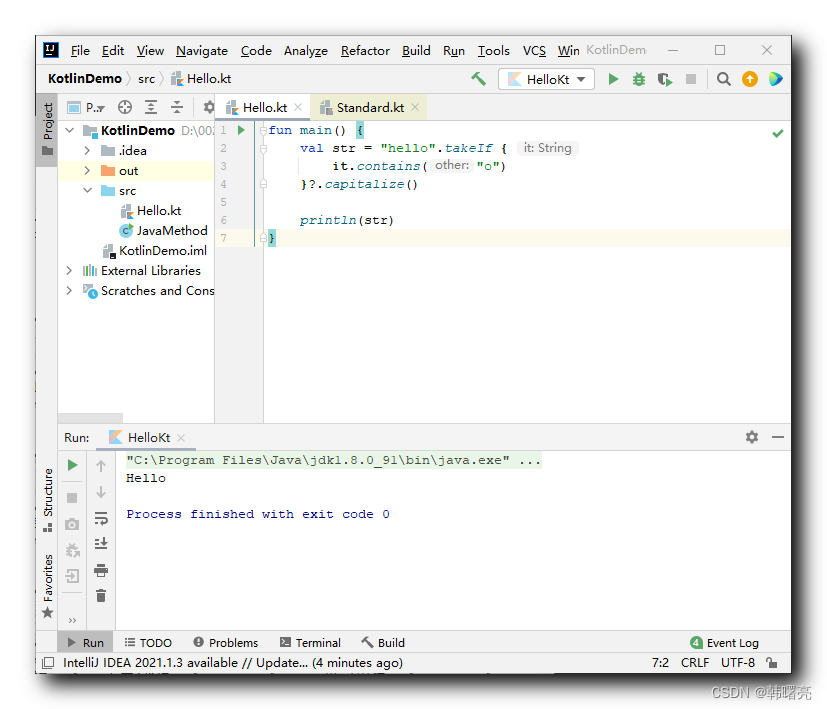# 七、takeUnless 标准库函数

takeUnless 函数 与 takeIf 函数 效果正好相反 ;

takeUnless 函数 的 返回值 由其 Lambda 表达式参数的返回值 确定 ,

Lambda 表达式 返回 false , 则 返回 接收者对象 ;

Lambda 表达式 返回 true , 则 返回 null 空值 ;

takeUnless 函数原型 :

``````/**
* 如果不满足给定的[谓词]，则返回' this '值;如果满足，则返回' null '值。
*
* 有关详细使用信息，请参阅[scope functions]的文档
* (https://kotlinlang.org/docs/reference/scope-functions.html#takeif-and-takeunless)。
*/
@kotlin.internal.InlineOnly
@SinceKotlin("1.1")
public inline fun <T> T.takeUnless(predicate: (T) -> Boolean): T? {

contract {

callsInPlace(predicate, InvocationKind.EXACTLY_ONCE)
}
return if (!predicate(this)) this else null
}
``````

`takeUnless` 函数的 Lambda 表达式 参数 返回的结果 为 `true` ,

``````fun main() {

val str = "hello".takeUnless {

it.contains("o")
}?.capitalize()

println(str)
}
``````

``````null
``````